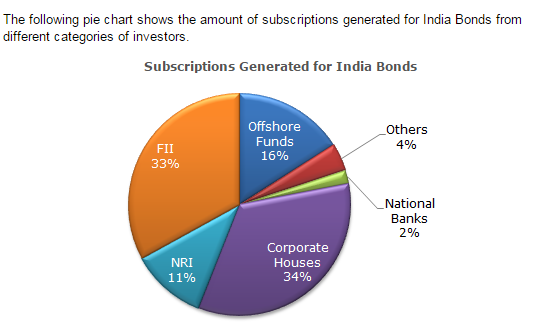Direction1.

In the corporate sector, approximately how many degrees should be there in the central angle ?

 A. 120 B. 121 C. 122 D. 123
Explanation: 34 x 3.6 = 122.4 (since 1% = 3.6 degrees)

2.

If the investment by NRI's are Rs 4,000 crore, then the investments by corporate houses and FII's together is:

 A. 24,000 crore B. 24,363 crore C. 25,423 crore D. 25,643 crore
Explanation: (67/11) x 4000 = 24 363.6364

3.

What percentage of the total investment is coming from FII's and NRI's ?

 A. 33 % B. 11 % C. 44 % D. 22 %
Explanation: (33 + 11) = 44

4.

If the total investment other than by FII and corporate houses is Rs 335,000 crore, then the investment by NRI's and Offshore funds will be (approximately) ?

 A. 274,100 B. 285,600 C. 293,000 D. Cannot be determined